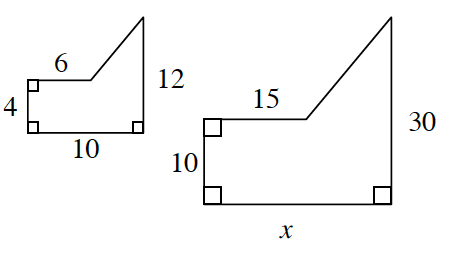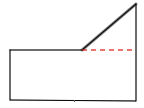### Home > GC > Chapter 6 > Lesson 6.1.2 > Problem6-15

6-15.The two shapes at right are similar.

1. Find the value of $x$. Show all work.

Set up a proportion to find the value of $x$.

2. Find the area of each shape.

Divide each shape into smaller, easier shapes to find the area.area of smaller figure: $56\text{ units}^²$
area of larger figure: $350\text{ units}^²$